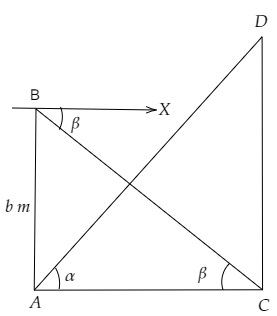# A tower subtends an angle $\alpha$ at a point A in the plane of its base and the angle of depression of the foot of the tower at a point b metres just above A is $\beta$. Prove that the height of the tower is $b \tan \alpha \cot \beta$.

Given:

A tower subtends an angle $\alpha$ at a point A  in the plane of its base and the angle of depression of the foot of the tower at a point b metres just above A  is $\beta$.

To do:

We have to prove that the height of the tower is $b \tan \alpha \cot \beta$.

Solution:Let $CD$ be the tower that subtends an angle $\alpha$ at a point A  in the plane of its base and the angle of depression of the foot of the tower at a point b metres just above A  is $\beta$.

From the figure,
$AB = b\ m, \angle \mathrm{DAC}=\alpha \mathrm{BCA}=\beta$

Let the height of the tower be $\mathrm{CD}=h\ m$ and $\mathrm{AC}=x\ m$

In $\Delta ADC$,

$\tan \theta=\frac{\text { Perpendicular }}{\text { Base }}$

$\tan \alpha=\frac{\mathrm{DC}}{\mathrm{AC}}$

$\tan \alpha=\frac{h}{x}$...........(i)

$\Rightarrow x=\frac{h}{\tan \alpha}$

Similarly,

In $\triangle \mathrm{ABC}$

$\tan \beta=\frac{\mathrm{AB}}{\mathrm{AC}}$

$=\frac{b}{x}$

$\Rightarrow x=\frac{b}{\tan \beta}$...........(ii)

From (i) and (ii), we get,

$\frac{h}{\tan \alpha}=\frac{b}{\tan \beta}$

$\Rightarrow h=b \frac{\tan \alpha}{\tan \beta}$

$=b \tan \alpha\cot \beta$

Hence proved.

Updated on: 10-Oct-2022

107 Views